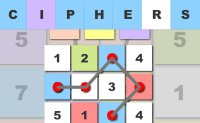Ciphers

 CiphersCiphers is a math matching game. On the top we see a number which needs to be summarized. In the grid above we use the 'mouse' to match the numbers which in addition gives us the correct sum. Therefore we need math skills and a bit of logic for the overview.

Tags:
puzzle